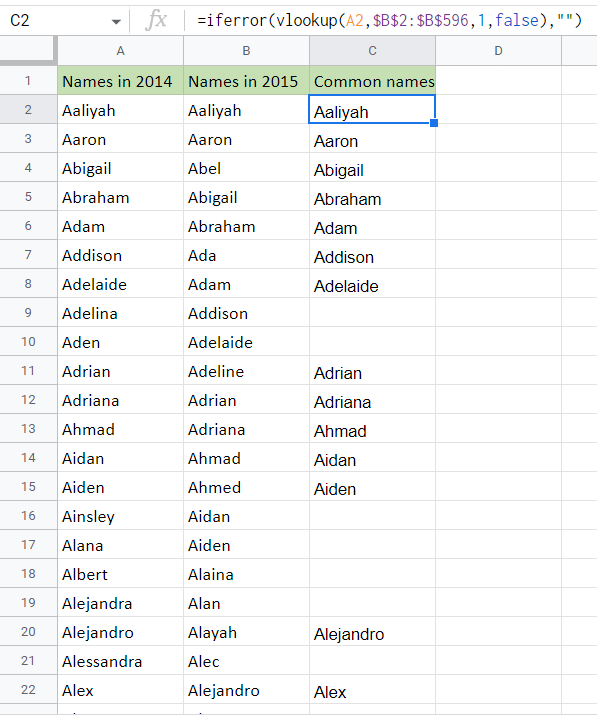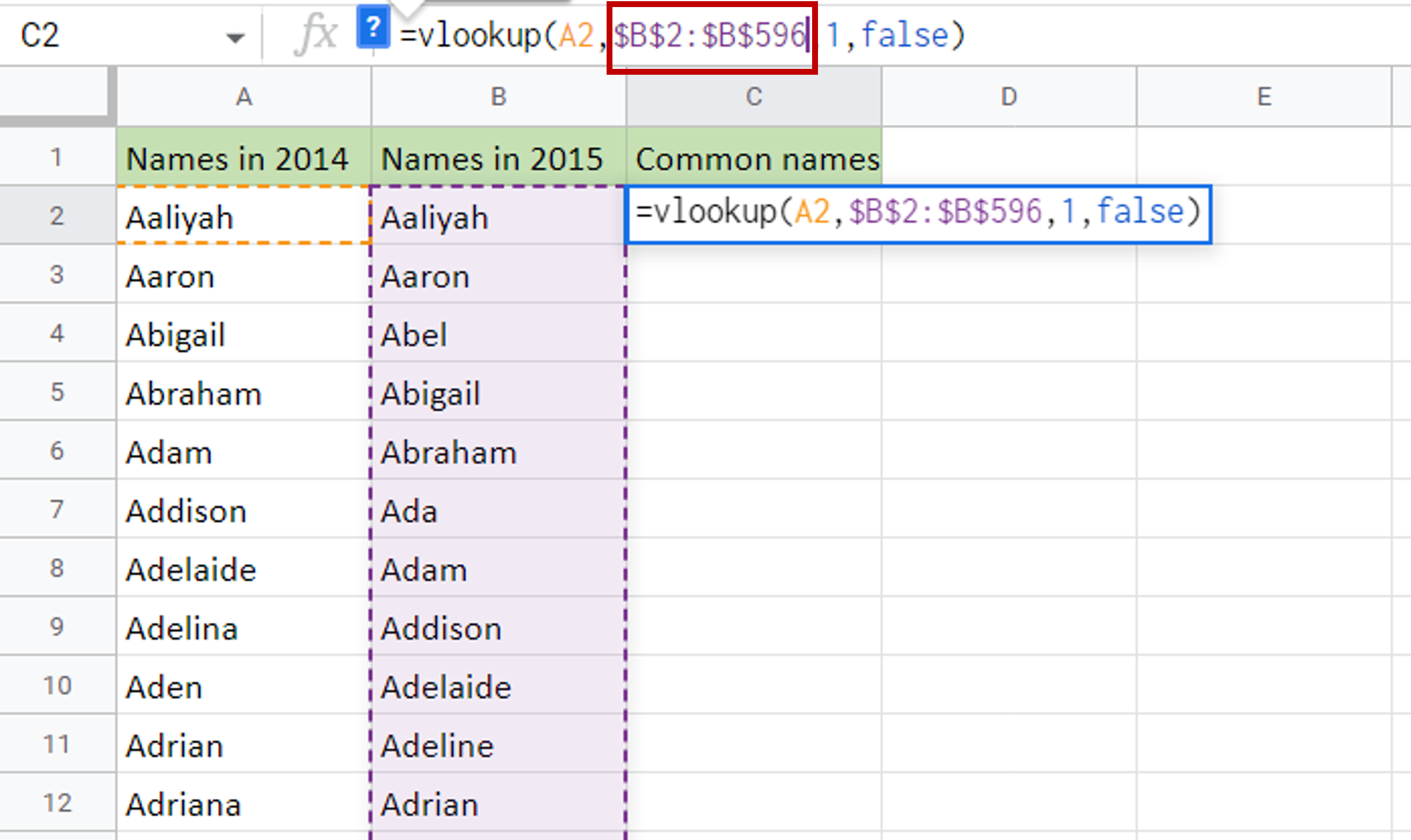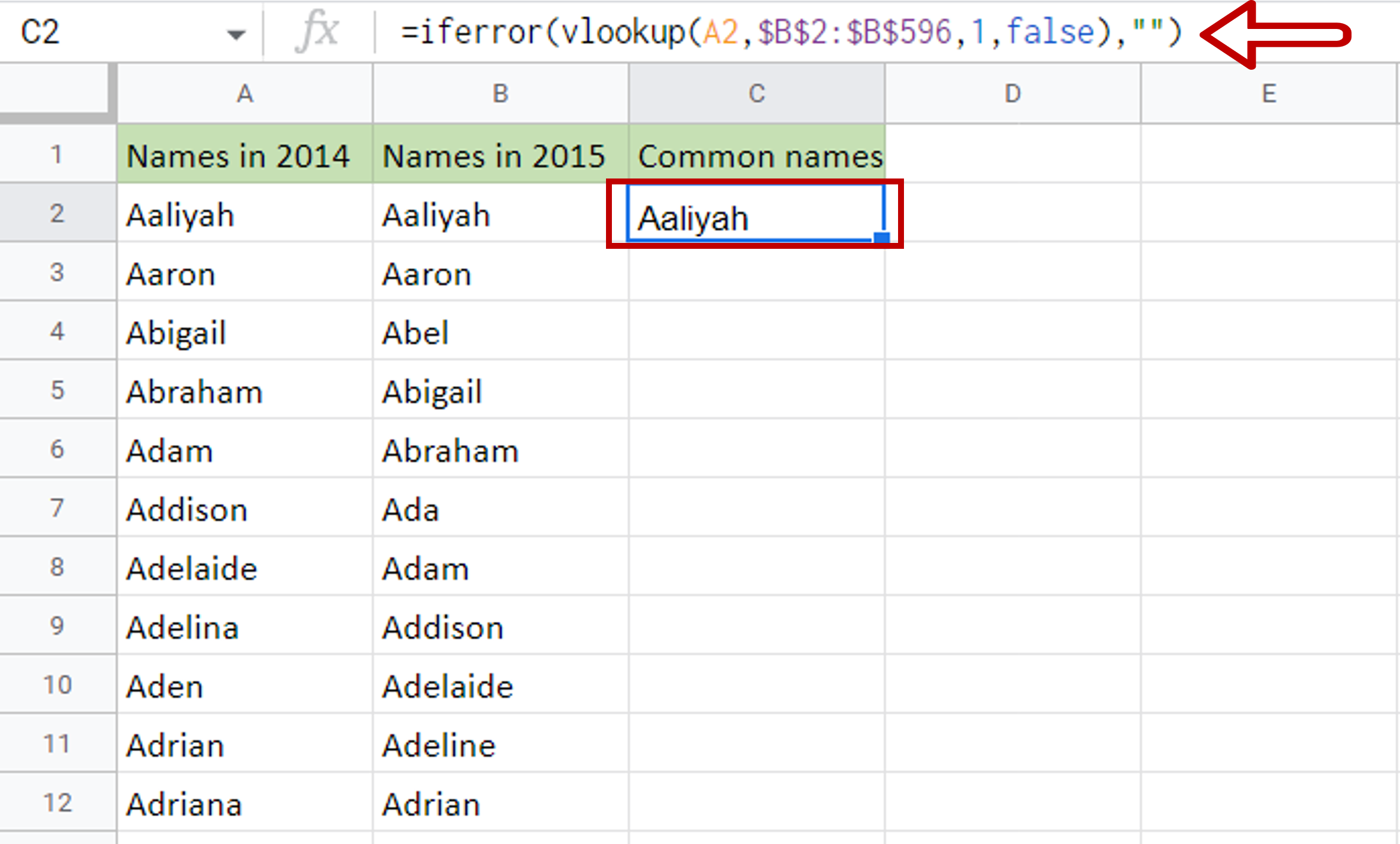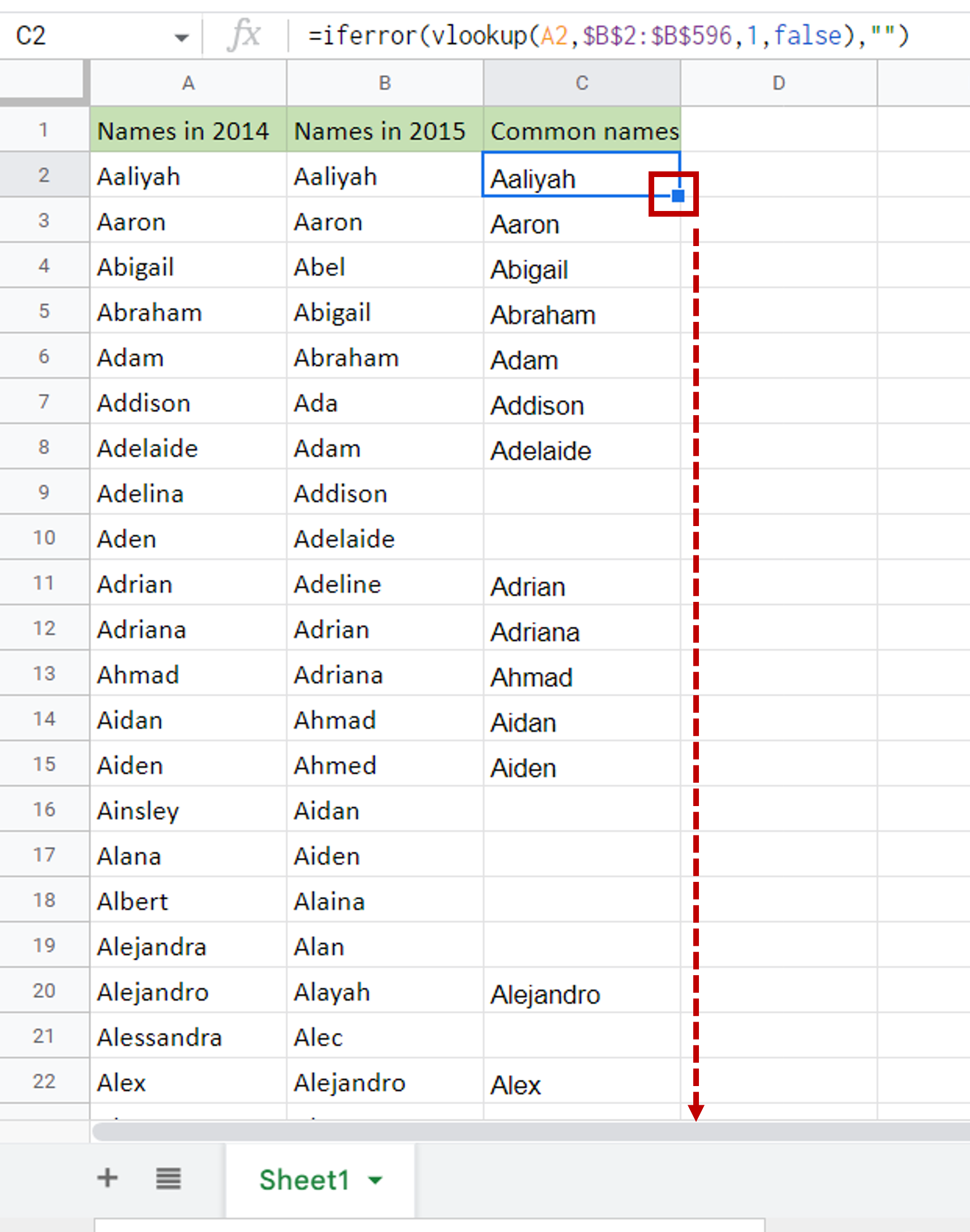# How to compare two columns in Google Sheets

You can watch a video tutorial here.

In Google Sheets, this can be done using the VLOOKUP() function.

1. VLOOKUP() function: this searches for a value in the leftmost column of a range, and returns the value in the column specified from the row of the match in the range.
1. Syntax: VLOOKUP(search_key, range, index, [is_sorted])
1. search_key: the cell reference of the value to search for
2. range: the range cells in which to search for the value
3. index: the index number of the column from which the found value is to be returned
4. is_sorted [optional]: TRUE will return an approximate match, FALSE will return an exact match

In this example, we will use VLOOKUP() to find names in the first column that match those in the second column.Comparing two columns of data is an operation you will frequently need to do when working with datasets. This happens especially when you are combining data from multiple sources and need to identify duplicates or matches.

### Step 1 – Create the formula– Select the cell where the result is to appear
– Type the formula using cell references:
=VLOOKUP(Names in 2014, \$range of Names in 2015\$, 1, FALSE)
– Since there is only one column, the index will be set to 1

### Step 2 – Make the range being looked up constant– Select the cell with the formula
– Press F2  to edit the cell
– Select the range of ‘Names in 2015’ and press F4
– This will add dollar signs (\$) to the cell references to make them constant

### Step 3 – Enhance the formula to handle errors i.e. missing values– If the lookup function cannot find a match in the second column i.e. ‘Names in 2015’, an error message is returned  – #N/A
– Change the formula to display an empty cell if a match cannot be found:
= IFERROR(<vlookup formula>,””)

### Step 4 – Copy the formula– Select the cell with the formula
– Place the pointer over the lower right corner of the cell, on the fill handle
– When the pointer becomes a cross, double-click
– The formula will be copied to the rest of the column

### Step 5 – Check the result– The ‘Common Names’ column has the list of names common between both columns
– The blank cells in the ‘Column Names’ column correspond to names in the ‘Names in 2014’ column that are not in ‘Names in 2015’
– To find names in ‘Names in 2015’ that are not in ‘Names in 2014’, create a new column and repeat the steps given above, using ‘Names in 2015’ as the search key and ‘Names in 2014’ as the range